Group scheme

(diff) ← Older revision | Latest revision (diff) | Newer revision → (diff)

A generalization of the concept of an algebraic group. Let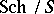be the category of schemes over a ground scheme; a group object of this category is known as a group scheme over the scheme(or a group-scheme, or an-scheme group). For a group schemeoverthe functor of points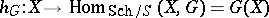is a contravariant functor from the categoryinto the category of groups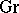. The categoryof group schemes overis defined as the complete subcategory of the category of such functors formed by the representable functors (cf. Representable functor).

Contents

Examples.

1) An algebraic group over a fieldis a reduced group scheme of finite type over. (A reduced group scheme of finite type over a field is sometimes referred to as an algebraic group.)

2) A functor which assigns to an-schemethe additive (or multiplicative) group of the ring of sections of the structure sheafis representable. The corresponding group scheme overis said to be the additive (or multiplicative) group scheme, and is denoted by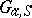(or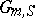). For any-scheme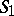one has3) Each abstract groupdefines a group scheme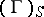, which is the direct sum of a family of schemes, each one of which is isomorphic to. The corresponding functor maps an-schemeto the direct sum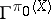, where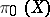is the set of connected components of.

Ifis a group scheme overthen, for any point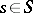, the fibre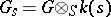is a group scheme over the residue fieldof this point. In particular, any group scheme of finite type overcan be regarded as a family of algebraic groups parametrized by the base. The terminology of the theory of schemes is extended to group schemes; thus, one speaks of smooth, flat, finite, and singular group schemes.

For any group schemethe corresponding reduced schemeis also a group scheme; the canonical closed imbedding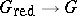is a morphism of group schemes. Each reduced group scheme of locally finite type over a perfect field is smooth. Each reduced group scheme of locally finite type over a field of characteristic zero is reduced (Cartier's theorem).

Many concepts and results in the theory of algebraic groups have their analogues for group schemes. Thus, there exists an analogue of the structure theory of Borel–Chevalley for affine algebraic groups , and a cohomology theory of extensions of group schemes and homogeneous spaces over group schemes has been developed , . On the other hand, many problems and results specific to the theory of group schemes are connected with the presence of nilpotent elements in the structure sheaf of both the ground scheme and the group scheme itself. Thus, infinitesimal and formal deformations of group schemes , problems of lifting into zero characteristic, and formal completion of group schemes (cf. Formal group) have all been studied. Group schemes arise in a natural manner in the study of algebraic groups over a field of positive characteristic (cf.-divisible group).

The concept of an affine group scheme over an affine ground schemeis dual to the concept of a commutative Hopf algebra; this is the case if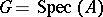is a group scheme for whichis a commutative Hopf algebra.

How to Cite This Entry:
Group scheme. Encyclopedia of Mathematics. URL: http://encyclopediaofmath.org/index.php?title=Group_scheme&oldid=18884
This article was adapted from an original article by I.V. Dolgachev (originator), which appeared in Encyclopedia of Mathematics - ISBN 1402006098. See original article